# Math in Focus Grade 7 Chapter 2 Lesson 2.5 Answer Key Operations with Rational Numbers

Go through the Math in Focus Grade 7 Workbook Answer Key Chapter 2 Lesson 2.5 Operations with Rational Numbers to finish your assignments.

## Math in Focus Grade 7 Course 2 A Chapter 2 Lesson 2.5 Answer Key Operations with Rational Numbers

### Math in Focus Grade 7 Chapter 2 Lesson 2.5 Guided Practice Answer Key

Copy and Complete

Question 1.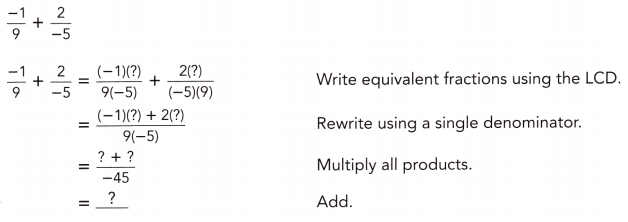–$$\frac{1}{9}$$ + $$\frac{2}{-5}$$
Write equivalent fractions using the LCD
$$\frac{(-1)(-5)}{(9)(-5)}$$ + $$\frac{2(9)}{(-5)(9)}$$
= $$\frac{5}{-45}$$ + $$\frac{18}{-45}$$
= $$\frac{5+18}{-45}$$
= $$\frac{23}{-45}$$

Question 2.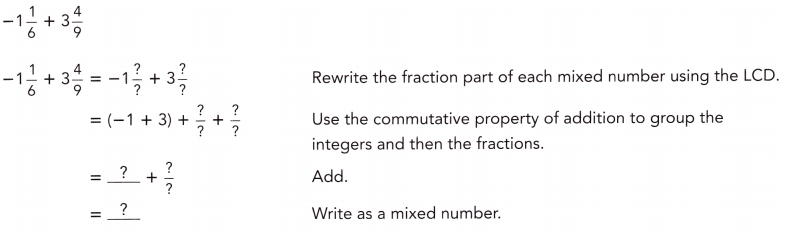-1 $$\frac{1}{6}$$ + 3$$\frac{4}{9}$$
= -1 – $$\frac{1}{6}$$ + 3 + $$\frac{4}{9}$$
= -1 + 3 = 2
= –$$\frac{1}{6}$$ + $$\frac{4}{9}$$
LCD of $$\frac{1}{6}$$ and $$\frac{4}{9}$$ is 18
= –$$\frac{3}{18}$$ + $$\frac{8}{18}$$ = $$\frac{5}{18}$$
2 + $$\frac{5}{18}$$ = 2$$\frac{5}{18}$$

Question 3.
$$\frac{1}{6}$$ + $$\left(\frac{-5}{9}\right)$$ + $$\left(\frac{-1}{3}\right)$$
Add two rational numbers at a time, working from left to right.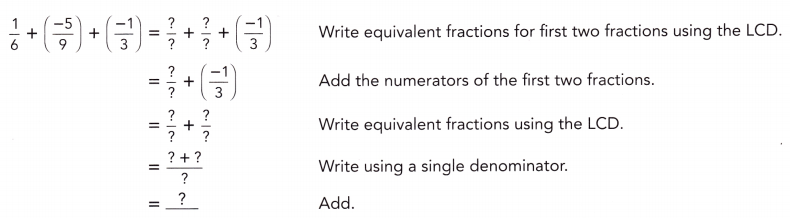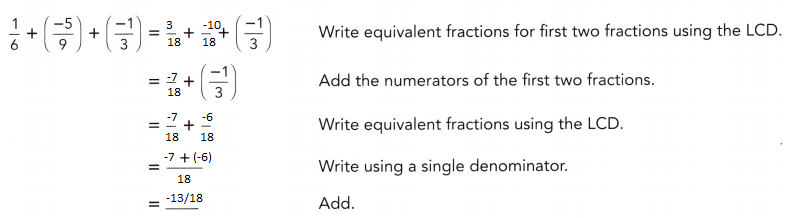Method 2
Use a common denominator for all three fractions.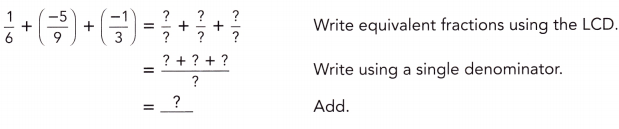$$\frac{1}{6}$$ + $$\left(\frac{-5}{9}\right)$$ + $$\left(\frac{-1}{3}\right)$$
= $$\frac{3}{18}$$ + $$\left(\frac{-10}{18}\right)$$ + $$\left(\frac{-6}{18}\right)$$
= (3 -10 – 6)/18
= -13/18

Evaluate each expression.

Question 4.
$$\frac{1}{4}$$ – $$\frac{3}{10}$$
The fractions have unlike denominators.
So, find the LCD for 1/4 and 3/10 that is 20.
$$\frac{1}{4}$$ × $$\frac{5}{5}$$ – $$\frac{3}{10}$$ × $$\frac{2}{2}$$
$$\frac{5}{20}$$ – $$\frac{6}{20}$$ = – $$\frac{1}{20}$$

Question 5.
$$\frac{7}{8}$$ – $$\frac{9}{10}$$
Given,
$$\frac{7}{8}$$ – $$\frac{9}{10}$$
The fractions have unlike denominators.
LCD of 8 and 10 is 40
$$\frac{7}{8}$$ × $$\frac{5}{5}$$ – $$\frac{9}{10}$$ × $$\frac{4}{4}$$
$$\frac{35}{40}$$ – $$\frac{36}{40}$$ = – $$\frac{1}{40}$$

Question 6.
3$$\frac{1}{4}$$ – 7$$\frac{5}{6}$$
Given
3$$\frac{1}{4}$$ – 7$$\frac{5}{6}$$
Rewriting the equation
3 + $$\frac{1}{4}$$ – 7 – $$\frac{5}{6}$$
3 – 7 = -4
Now subtract the fractions
$$\frac{1}{4}$$ – $$\frac{5}{6}$$
LCM of 4 and 6 is 12
$$\frac{1}{4}$$ × $$\frac{3}{3}$$ – $$\frac{5}{6}$$ × $$\frac{2}{2}$$
$$\frac{3}{12}$$ – $$\frac{10}{12}$$ = – $$\frac{7}{12}$$
-4 – $$\frac{7}{12}$$ = -4$$\frac{7}{12}$$

Question 7.
$$\frac{3}{7}$$ – $$\frac{27}{28}$$ – $$\frac{3}{14}$$
Given
$$\frac{3}{7}$$ – $$\frac{27}{28}$$ – $$\frac{3}{14}$$
LCM of 7, 14, 28 is 28.
$$\frac{3}{7}$$ × $$\frac{4}{4}$$ – $$\frac{27}{28}$$ × $$\frac{1}{1}$$ – $$\frac{3}{14}$$ × $$\frac{2}{2}$$
$$\frac{12}{28}$$ – $$\frac{27}{28}$$ – $$\frac{6}{28}$$
= (12 – 27 – 6)/28
= -21/28
= -3/4

Solve.

Question 8.
Philadelphia suffered a severe snowstorm in 1996 that left 30$$\frac{7}{10}$$ inches of snow on the ground. Another severe snowstorm occured in 2010, when 28$$\frac{1}{2}$$ inches of snow fell.
a) Write a subtraction expression for the difference in depth of these two record snowfalls.
30$$\frac{7}{10}$$ – 28$$\frac{1}{2}$$

b) Rewrite the expression as an addition expression.
Answer: – 28$$\frac{1}{2}$$ + 30$$\frac{7}{10}$$

c) Find the difference in these two record snowfalls.
30$$\frac{7}{10}$$ – 28$$\frac{1}{2}$$
Rewriting the equation
30 + $$\frac{7}{10}$$ – 28 – $$\frac{1}{2}$$
30 – 28 = 2
$$\frac{7}{10}$$ – $$\frac{1}{2}$$
LCD = 10
$$\frac{7}{10}$$ – $$\frac{5}{10}$$ = $$\frac{2}{10}$$ = $$\frac{1}{5}$$
2 + $$\frac{1}{5}$$ = 2 $$\frac{1}{5}$$

Copy and Complete.

Question 9.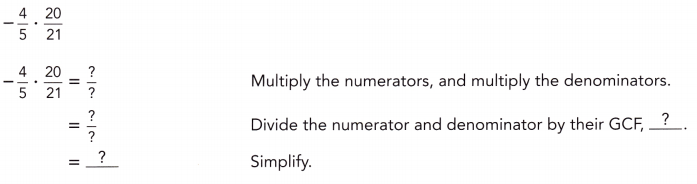–$$\frac{4}{5}$$ × $$\frac{20}{21}$$
Multiply the numerators and multiply the denominators
= (-4 × 20)/(5 × 21)
= –$$\frac{80}{105}$$

Question 10.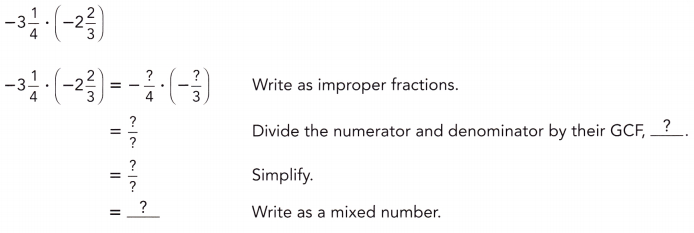-3$$\frac{1}{4}$$ × -2$$\frac{2}{3}$$
Write as improper fractions
–$$\frac{13}{4}$$ × –$$\frac{8}{3}$$
= $$\frac{104}{12}$$
= $$\frac{52}{6}$$
= $$\frac{26}{3}$$
= 8$$\frac{2}{3}$$

Evaluate each quotient.

Question 11.
$$\frac{3}{20}$$ ÷ $$\left(-\frac{6}{35}\right)$$
Dividing two fractions is the same as multiplying the first fraction by the reciprocal of the second fraction.
$$\frac{3}{20}$$ × –$$\frac{35}{6}$$
= (3 × -35)/(20 × 6)
= -105/120
= -7/8

Question 12.
-3$$\frac{1}{3}$$ ÷ $$\left(-1 \frac{1}{4}\right)$$
Given,
-3$$\frac{1}{3}$$ ÷ $$\left(-1 \frac{1}{4}\right)$$
$$\frac{10}{3}$$ ÷ $$\frac{5}{4}$$
LCD of 3 and 4 is 12.
$$\frac{10}{3}$$ × $$\frac{4}{4}$$ ÷ $$\frac{5}{4}$$ × $$\frac{3}{3}$$
$$\frac{40}{12}$$ ÷ $$\frac{15}{12}$$
$$\frac{40}{12}$$ = 2 $$\frac{2}{3}$$

Question 13.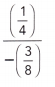Dividing two fractions is the same as multiplying the first fraction by the reciprocal of the second fraction.
$$\frac{1}{4}$$ ÷ $$\frac{-3}{8}$$ = $$\frac{-2}{3}$$

Question 14.
A pancake recipe requires 1$$\frac{2}{3}$$ cups of flour to make 20 pancakes and you have 9 cups of flour.
a) How many pancakes can you make with 1 cup of flour?
Given,
A pancake recipe requires 1$$\frac{2}{3}$$ cups of flour to make 20 pancakes
Let 1 cup of flour is x
1 $$\frac{2}{3}$$ x = 20
x = 12

b) How many pancakes can you make with 9 cups of flour?
1 cup = 12 pancakes
9 cups = 9 × 12 = 108
Thus you can make 81 pancakes with 9 cups of flour.

c) Do you have enough to make 100 pancakes? Explain your reasoning.
1 cup of flour = 12 pancakes
9 cups = 9 × 12 = 108
Thus you can make 81 pancakes with 9 cups of flour.
As I can make 108 pancakes with 9 cups of flour, I can conclude that I have enough flour to make 100 pancakes

### Math in Focus Course 2A Practice 2.5 Answer Key

Question 1.
$$\frac{1}{2}$$ + $$\left(-\frac{5}{6}\right)$$
Given,
$$\frac{1}{2}$$ + $$\left(-\frac{5}{6}\right)$$
The fractions of the denominators are not the same. So, we have to find the LCD of the denominators and rewrite the fractions.
$$\frac{1}{2}$$ – $$\frac{5}{6}$$
LCD of 2 and 6 is 6.
$$\frac{1}{2}$$ × $$\frac{3}{3}$$ – $$\frac{5}{6}$$ × $$\frac{1}{1}$$
$$\frac{3}{6}$$ – $$\frac{5}{6}$$ = – $$\frac{2}{6}$$ or – $$\frac{1}{3}$$

Question 2.
–$$\frac{6}{7}$$ + $$\frac{3}{14}$$
Given,
–$$\frac{6}{7}$$ + $$\frac{3}{14}$$
The fractions of the denominators are not the same. So, we have to find the LCD of the denominators and rewrite the fractions.
LCD of 7 and 14 is 14.
–$$\frac{12}{14}$$ + $$\frac{3}{14}$$ = –$$\frac{9}{14}$$

Question 3.
–$$\frac{1}{7}$$ + $$\left(\frac{-3}{5}\right)$$
Given,
–$$\frac{1}{7}$$ + $$\left(\frac{-3}{5}\right)$$
The fractions of the denominators are not the same. So, we have to find the LCD of the denominators and rewrite the fractions.
-($$\frac{1}{7}$$ + $$\frac{3}{5}$$)
LCD of 7 and 5 is 35
-($$\frac{5}{35}$$ + $$\frac{21}{35}$$)
–$$\frac{26}{35}$$

Question 4.
$$\frac{1}{2}$$ + $$\left(-\frac{2}{5}\right)$$ + $$\frac{1}{4}$$
Given,
$$\frac{1}{2}$$ + $$\left(-\frac{2}{5}\right)$$ + $$\frac{1}{4}$$
The fractions of the denominators are not the same. So, we have to find the LCD of the denominators and rewrite the fractions.
$$\frac{1}{2}$$ + $$\frac{1}{4}$$ – $$\frac{2}{5}$$
$$\frac{3}{4}$$ – $$\frac{2}{5}$$
LCD of 4 and 5 is 20.
$$\frac{15}{20}$$ – $$\frac{8}{20}$$ = $$\frac{7}{20}$$

Question 5.
–$$\frac{1}{7}$$ + $$\left(\frac{-5}{6}\right)$$ + $$\left(\frac{-1}{3}\right)$$
Given,
–$$\frac{1}{7}$$ + $$\left(\frac{-5}{6}\right)$$ + $$\left(\frac{-1}{3}\right)$$
The fractions of the denominators are not the same. So, we have to find the LCD of the denominators and rewrite the fractions.
–$$\frac{1}{7}$$ – $$\frac{5}{6}$$ – $$\frac{1}{3}$$
– ($$\frac{1}{7}$$ + $$\frac{5}{6}$$ + $$\frac{1}{3}$$ )
LCD of 7, 6 and 3 is 42.
– ($$\frac{6}{42}$$ + $$\frac{35}{42}$$ + $$\frac{14}{42}$$)
– (6 + 35 + 14)/42
– $$\frac{55}{42}$$

Question 6.
$$\frac{3}{5}$$ – $$\frac{2}{3}$$
Given,
$$\frac{3}{5}$$ – $$\frac{2}{3}$$
The fractions of the denominators are not the same. So, we have to find the LCD of the denominators and rewrite the fractions.
LCD of 5 and 3 is 15.
$$\frac{9}{15}$$ – $$\frac{10}{15}$$ = – $$\frac{1}{15}$$

Question 7.
–$$\frac{1}{7}$$ – $$\frac{3}{14}$$
Given,
–$$\frac{1}{7}$$ – $$\frac{3}{14}$$
The fractions of the denominators are not the same. So, we have to find the LCD of the denominators and rewrite the fractions.
LCD of 7 and 14 is 14.
-($$\frac{1}{7}$$ + $$\frac{3}{14}$$)
-($$\frac{2}{14}$$ + $$\frac{3}{14}$$)
– $$\frac{5}{14}$$

Question 8.
–$$\frac{1}{5}$$ – $$\left(\frac{-2}{7}\right)$$
Given,
–$$\frac{1}{5}$$ – $$\left(\frac{-2}{7}\right)$$
The fractions of the denominators are not the same. So, we have to find the LCD of the denominators and rewrite the fractions.
– $$\frac{1}{5}$$ + $$\frac{2}{7}$$
LCD of 7 and 5 is 35
-($$\frac{7}{35}$$ + $$\frac{10}{35}$$)
–$$\frac{17}{35}$$

Question 9.
$$\frac{1}{3}$$ – $$\left(-\frac{2}{5}\right)$$ – $$\frac{3}{4}$$
Given,
$$\frac{1}{3}$$ – $$\left(-\frac{2}{5}\right)$$ – $$\frac{3}{4}$$
The fractions of the denominators are not the same. So, we have to find the LCD of the denominators and rewrite the fractions.
$$\frac{1}{3}$$ + $$\frac{2}{5}$$ – $$\frac{3}{4}$$
LCD of 3, 5, and 7 is 60.
$$\frac{20}{60}$$ + $$\frac{24}{60}$$ – $$\frac{45}{60}$$
$$\frac{44}{60}$$ – $$\frac{45}{60}$$ = – $$\frac{1}{60}$$

Question 10.
–$$\frac{7}{25}$$ • $$\frac{5}{14}$$
Given,
–$$\frac{7}{25}$$ • $$\frac{5}{14}$$
-(7 × 5)/(25 × 14)
= -1/10

Question 11.
$$\frac{5}{8}$$ • $$\left(-\frac{4}{15}\right)$$
Given,
$$\frac{5}{8}$$ • $$\left(-\frac{4}{15}\right)$$
(5 × -4)/(8 × 15)
= -20/120
= -1/6

Question 12.
$$\frac{7}{30}$$ • $$\left(-\frac{6}{7}\right)$$
Given,
$$\frac{7}{30}$$ • $$\left(-\frac{6}{7}\right)$$
(7 × -6)/(30 × 7)
= -1/5

Question 13.
–$$\frac{8}{27}$$ • $$\left(-\frac{9}{40}\right)$$
Given,
–$$\frac{8}{27}$$ • $$\left(-\frac{9}{40}\right)$$
= (-8 × -9)/(27 × 40)
= 1/15

Question 14.
–$$\frac{11}{16}$$ • $$\left(-\frac{4}{33}\right)$$
Given,
–$$\frac{11}{16}$$ • $$\left(-\frac{4}{33}\right)$$
(-11 × -4)/(16 × 33)
1/12

Question 15.
$$\frac{5}{8}$$ • $$\left(-2 \frac{4}{5}\right)$$
Given,
$$\frac{5}{8}$$ • $$\left(-2 \frac{4}{5}\right)$$
$$\frac{5}{8}$$ • $$\left(\frac{-6}{5}\right)$$
(5 × -6)/(8 × 5)
-3/4

Question 16.
–$$\frac{3}{22}$$ • 1$$\frac{5}{6}$$
Given,
–$$\frac{3}{22}$$ • 1$$\frac{5}{6}$$
Convert from mixed fraction to the improper fraction to find the product of two fractions.
–$$\frac{3}{22}$$ • $$\frac{11}{6}$$
= –$$\frac{3×11}{22×6}$$
= –$$\frac{33}{132}$$
= –$$\frac{1}{4}$$

Question 17.
3$$\frac{1}{8}$$ • $$\left(-\frac{3}{10}\right)$$
Given,
3$$\frac{1}{8}$$ • $$\left(-\frac{3}{10}\right)$$
Convert from mixed fraction to the improper fraction to find the product of two fractions.
$$\frac{25}{8}$$ • –$$\frac{3}{10}$$
= –$$\frac{75}{80}$$
= –$$\frac{15}{16}$$

Question 18.
-4$$\frac{1}{2}$$ • $$\left(-1 \frac{8}{9}\right)$$
Given,
-4$$\frac{1}{2}$$ • $$\left(-1 \frac{8}{9}\right)$$
Convert from mixed fraction to the improper fraction to find the product of two fractions.
–$$\frac{9}{2}$$ • –$$\frac{17}{9}$$
minus × minus = plus
We get
$$\frac{9}{2}$$ • $$\frac{17}{9}$$
= $$\frac{51}{6}$$
= $$\frac{17}{2}$$

Question 19.
-10 ÷ $$\left(-\frac{5}{6}\right)$$
Given,
-10 ÷ $$\left(-\frac{5}{6}\right)$$
First cancel the negative signs
We get
10 ÷ $$\frac{5}{6}$$
(10 × 6)/(1 × 5)
= 60/5
= 12

Question 20.
$$\frac{9}{25}$$ ÷ (-18)
Given,
$$\frac{9}{25}$$ ÷ (-18)
(9 × 1)/25 × -28
=$$\frac{9}{-450}$$
= –$$\frac{1}{50}$$

Question 21.
–$$\frac{3}{8}$$ ÷ $$\left(-\frac{1}{8}\right)$$
Given,
–$$\frac{3}{8}$$ ÷ $$\left(-\frac{1}{8}\right)$$
First cancel the negative signs
We get
$$\frac{3}{8}$$ ÷ $$\frac{1}{8}$$ = 3

Question 22.
–$$\frac{1}{4}$$ ÷ $$\frac{3}{8}$$
Given,
–$$\frac{1}{4}$$ ÷ $$\frac{3}{8}$$
= -(1 × 8)/(4 × 3) = -8/12
= -2/3

Question 23.
$$\frac{5}{12}$$ ÷ $$\left(-\frac{1}{6}\right)$$
Given,
$$\frac{5}{12}$$ ÷ $$\left(-\frac{1}{6}\right)$$
(5 × 6)/(-12 × 1)
= -30/12
= -5/2
= $$\left(-2 \frac{1}{2}\right)$$

Question 24.
-1$$\frac{1}{4}$$ ÷ $$\frac{3}{4}$$
Given,
-1$$\frac{1}{4}$$ ÷ $$\frac{3}{4}$$
Convert from mixed fraction to the improper fraction
–$$\frac{5}{4}$$ ÷ $$\frac{3}{4}$$
= -(5 × 4)/(4 × 3)
= –$$\frac{20}{12}$$
= -1$$\frac{2}{3}$$

Question 25.
$$\frac{8}{15}$$ ÷ $$\left(-2 \frac{2}{3}\right)$$
Given,
$$\frac{8}{15}$$ ÷ $$\left(-2 \frac{2}{3}\right)$$
Convert from mixed fraction to the improper fraction
$$\frac{8}{15}$$ ÷ –$$\frac{8}{3}$$
= (8 × 3)/(15 × -8)
= –$$\frac{1}{5}$$

Question 26.
3$$\frac{3}{4}$$ ÷ $$\left(-\frac{1}{4}\right)$$
Given,
3$$\frac{3}{4}$$ ÷ $$\left(-\frac{1}{4}\right)$$
Convert from mixed fraction to the improper fraction
$$\frac{15}{4}$$ ÷ –$$\frac{1}{4}$$
= (15 × 4)/(4 × -1)
= –$$\frac{60}{4}$$
= -15

Question 27.
2$$\frac{1}{2}$$ ÷ $$\left(-1 \frac{2}{3}\right)$$
Given,
2$$\frac{1}{2}$$ ÷ $$\left(-1 \frac{2}{3}\right)$$
Convert from mixed fraction to the improper fraction
$$\frac{5}{2}$$ ÷ –$$\frac{5}{3}$$
= -(5 × 3)/(2 × 5)
= –$$\frac{3}{2}$$ or -1$$\frac{1}{2}$$

Question 28.
-2$$\frac{2}{7}$$ ÷ $$\left(-1 \frac{3}{7}\right)$$
Given,
-2$$\frac{2}{7}$$ ÷ $$\left(-1 \frac{3}{7}\right)$$
Convert from mixed fraction to the improper fraction
–$$\frac{16}{7}$$ ÷  –$$\frac{10}{7}$$
Cancel the negative signs and then perform division operation
$$\frac{16}{7}$$ ÷ $$\frac{10}{7}$$
= (16 × 7)/(7 × 10)
= $$\frac{8}{5}$$
= 1 $$\frac{3}{5}$$

Question 29.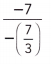Given,-7 ÷ –$$\frac{7}{3}$$
Cancel the negative signs and then perform division operation
(7 × 3)/(7)
= 21/7
= 3

Question 30.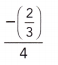Given,–$$\frac{2}{3}$$ ÷ 4
= -(2 × 1)/(3 × 4)
= -2/12
= –$$\frac{1}{6}$$

Question 31.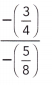Given,Cancel the negative signs and then perform division operation
$$\frac{3}{4}$$ ÷ $$\frac{5}{8}$$
= (3 × 8)/(4 × 5)
= 24/20
= 1$$\frac{1}{5}$$

Question 32.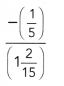Given,–$$\frac{1}{5}$$ ÷ 1$$\frac{2}{15}$$
–$$\frac{1}{5}$$ ÷ $$\frac{17}{15}$$
= -(1 × 15)/(5 × 17)
= –$$\frac{3}{17}$$

Question 33.
David hiked 15$$\frac{9}{10}$$ miles on Saturday and 6$$\frac{7}{10}$$ miles on Sunday. How much farther did David bike on Saturday than on Sunday?
David hiked 15$$\frac{9}{10}$$ miles on Saturday and 6$$\frac{7}{10}$$ miles on Sunday.
15$$\frac{9}{10}$$ – 6$$\frac{7}{10}$$ = 9 $$\frac{1}{5}$$

Question 34.
A recipe calls for $$\frac{3}{4}$$ cup of flour, but Kelli has only $$\frac{1}{4}$$ cup of flour. How much more flour does she need?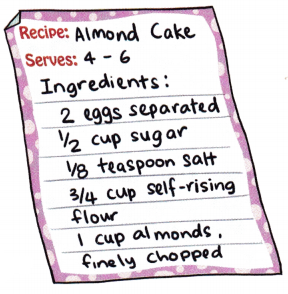Given,
A recipe calls for $$\frac{3}{4}$$ cup of flour, but Kelli has only $$\frac{1}{4}$$ cup of flour.
$$\frac{3}{4}$$ – $$\frac{1}{4}$$ = $$\frac{1}{2}$$
Thus Kelli needs $$\frac{1}{2}$$ cup of flour.

Question 35.
A weather report showed that the rainfall in Janesville was 2$$\frac{2}{3}$$ inches during the first half of January. At the end of January, the total rainfall was 3$$\frac{1}{4}$$ inches. How much did it rain in the second half of January?
Given,
A weather report showed that the rainfall in Janesville was 2$$\frac{2}{3}$$ inches during the first half of January.
At the end of January, the total rainfall was 3$$\frac{1}{4}$$ inches.
3$$\frac{1}{4}$$ – 2$$\frac{2}{3}$$
Convert from mixed fraction to the improper fraction
$$\frac{13}{4}$$ – $$\frac{8}{3}$$
LCD of 4 and 3 is 12
$$\frac{39}{12}$$ – $$\frac{32}{12}$$ = $$\frac{7}{12}$$

Question 36.
The sum of two rational numbers is 5$$\frac{1}{2}$$. If one of the numbers is 6$$\frac{3}{14}$$, find the other number.
The sum of two rational numbers is 5$$\frac{1}{2}$$. If one of the numbers is 6$$\frac{3}{14}$$
x + 6$$\frac{3}{14}$$ = 5$$\frac{1}{2}$$
x = 5$$\frac{1}{2}$$ – 6$$\frac{3}{14}$$
x = 5 + $$\frac{1}{2}$$ – 6 – $$\frac{3}{14}$$
x = -1 + $$\frac{2}{7}$$ = –$$\frac{5}{7}$$

Question 37.
MathJournal Peter adds $$\frac{1}{a}$$ + $$\left(-\frac{1}{b}\right)$$ and says the answer is $$\frac{1}{a-b}$$. Give an example to show that Peter is wrong.
$$\frac{1}{a}$$ + $$\left(-\frac{1}{b}\right)$$
$$\frac{1}{a}$$ – $$\frac{1}{b}$$
LCD of a and b is ab
$$\frac{b}{ab}$$ – $$\frac{a}{ab}$$
$$\frac{b-a}{ab}$$
By this we can say that Peter is wrong.

Question 38.
MathJournal Jo multiplies two mixed numbers, -4$$\frac{3}{5}$$ and 1$$\frac{2}{7}$$ as follows: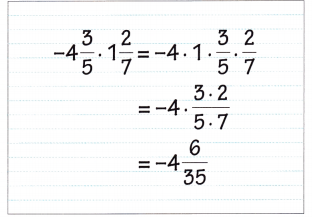Describe Jo’s mistakes. What is the correct answer?
-4$$\frac{3}{5}$$ × 1$$\frac{2}{7}$$
Convert from mixed fraction to the improper fraction
–$$\frac{23}{5}$$ × $$\frac{9}{7}$$
–$$\frac{207}{35}$$
-5$$\frac{32}{35}$$

Question 39.
A clock’s battery is running low. Every 6 hours, it slows down by $$\frac{1}{2}$$ hour. By how much does it slow down in 1 hour?
If its running low and every six hours it slows down half an hour, we need to divide 6 from 1/2.
6 divided by 1/2 is 12
It will slow down 12 minutes every hour.

Question 40.
A weighs 5$$\frac{1}{2}$$ pounds and package B weighs 1$$\frac{1}{4}$$ pounds. Find the average weight of the two packages.
Given,
A weighs 5$$\frac{1}{2}$$ pounds and package B weighs 1$$\frac{1}{4}$$ pounds.
5$$\frac{1}{2}$$ + 1$$\frac{1}{4}$$ = 6 $$\frac{3}{4}$$
Convert from mixed fraction to the improper fraction
6 $$\frac{3}{4}$$ = $$\frac{27}{4}$$
Average of two packets
$$\frac{27}{4}$$ ÷ 2 = $$\frac{27}{8}$$ = 3 $$\frac{3}{8}$$

Question 41.
A scientist measured the weight of some damp soil. After exposing the soil to the air for 4$$\frac{1}{2}$$ weeks, the scientist found that the weight had decreased by 5$$\frac{1}{8}$$ ounces. Find the average weight loss per week.
Let the weight loss per week is x
A scientist measured the weight of some damp soil. After exposing the soil to the air for 4$$\frac{1}{2}$$ weeks, the scientist found that the weight had decreased by 5$$\frac{1}{8}$$ ounces.
4$$\frac{1}{2}$$ weeks = 5$$\frac{1}{8}$$
1 week = x
x × 4$$\frac{1}{2}$$ = 5$$\frac{1}{8}$$
x = 5$$\frac{1}{8}$$ ÷ 4$$\frac{1}{2}$$
x = $$\frac{41}{8}$$ ÷ $$\frac{9}{2}$$
x = 1 $$\frac{5}{36}$$ ounces

Question 42.
A plank measures 4$$\frac{3}{4}$$ feet. Elizabeth cuts off $$\frac{2}{5}$$ of the plank. How long is the plank now?
A plank measures 4$$\frac{3}{4}$$ feet.
Elizabeth cuts off $$\frac{2}{5}$$ of the plank.
4$$\frac{3}{4}$$ – $$\frac{2}{5}$$ = 4 $$\frac{7}{20}$$
Thus the plank is 4 $$\frac{7}{20}$$ feet long now.